# 4D Shapes & 4D Geometry: Exploring Higher Dimensions

Posted by Randall Morgan on

# 4D Shapes & 4D Geometry"Higher dimensions are not just mathematical abstractions, they are a fundamental part of the universe we live in." - Michio Kaku

## 4D Shapes

In simple terms, four-dimensional geometry is the study of shapes and objects in a four-dimensional space. This is a space that has four dimensions, rather than the three dimensions that we are familiar with in our everyday lives (length, width, and height).

One way to think about four-dimensional space is to consider the three dimensions that we are familiar with as a kind of "slice" or "slice of bread" in a larger, four-dimensional "loaf of bread." Just as we can move around in three-dimensional space by moving forward, backward, left, right, up, and down, we can also move around in four-dimensional space by moving in a fourth direction, often referred to as "w" or "time."

In four-dimensional space, we can have four-dimensional shapes, such as a four-dimensional sphere or a four-dimensional cube. These shapes can be difficult to visualize, but we can try to imagine them by thinking about how they would look if we were able to "slice" through them and see the cross-sections in three-dimensional space.

Four-dimensional geometry is a fascinating and complex subject that has many interesting applications, including in physics and mathematics. However, it can also be challenging to wrap our heads around because it involves concepts that are beyond our everyday experience.The concept of four dimensions first began with a French mathematician,  physicist and philosopher - Jean le Rond d'Alembert. His book "Dimensions" was published in 1754.

Since then much work has been done exploring the incredible complexity of 4D geometry. Four dimensional space is much more complex than 3D space. We know that there are 5 regular polyhedral in three dimensions, each consisting of regular polygons - known as the 5 Platonic solids.### The six regular convex polychora in four dimensions"The concept of four-dimensional space is as beautiful as it is hard to imagine." -Albert Einstein

"There are sixteen regular polychora, six of which are convex (Wells 1986, p. 68) and ten of which are stellated (Wells 1991, p. 209). The regular convex polychora have four principal types of symmetry axes, and the projections into three-spaces orthogonal to these may be called the canonical projections." (Weisstein, Eric W. "Regular Polychoron.")

In four dimensions - there are six regular convex polychora, five of these can be thought of as 4D analogues of the platonic solids. Lets have a look at the incredible complexity and symmetry found in these 4D shapes.

## 4D Geometry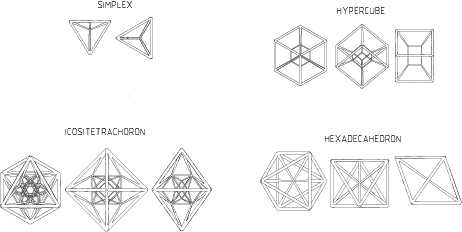There are only six regular convex 4-polytopes because of the constraints that are imposed by the rules of regular convexity. In order for a 4-polytope to be regular, it must satisfy certain conditions, such as having congruent regular faces, and having a symmetric arrangement of vertices.

It turns out that there are only six combinations of regular polygons that can be used to create regular convex 4-polytopes. These six combinations are:

• The 4-simplex, which is bounded by 5 tetrahedron faces.
• The tesseract, or 4-cube, which is bounded by 8 cube faces.
• The 16-cell, which is bounded by 16 tetrahedron faces.
• The 120-cell, which is bounded by 120 tetrahedron faces.
• The 600-cell, which is bounded by 600 tetrahedron faces.
• The 24-cell, which is bounded by 24 octahedron faces.

These are the only six regular convex 4-polytopes that can be constructed in four dimensions, and they are known as the regular convex 4-polychora. Each of these shapes has its own unique properties and symmetries, and they are important objects of study in mathematics and physics.

### 5-cell (Simplex)The 4-simplex is the simplest possible four dimensional shape. The regular 5-cell is bounded by 5 regular tetrahedra. The 5-cell is created by adding a point one edge length distance from all the other vertices - this cannot be done in 3 dimensions.

Below is the 5-cell performing a simple rotation in 3D.Details of the 5-cell

• 10 edges
• 10 faces
• 5 vertices
• 5 cells

### 8-cell (Hypercube)The hypercube, also known as a tesseract is a four dimensional cube. It is known as a measure polytope since it can be used to tile space in four dimensions - similar to how we measure volume in cubic units. This hyper-surface consists of 8 cubical cells.

The term tesseract is derived from the Greek word "téssara" meaning four and "aktís" meaning ray, referring to the four edges from each vertex.

Below is the Tesseract performing a simple rotation in 3D.Details of the 8-cell (Tesseract)

• 8 cells
• 24 faces
• 32 edges
• 16 verticesThe 16-cell, also known as the hexadecachoron or hexdecahedroid is the daul of the 8-cell. It was first described by Swiss mathematician Ludwig Schläfli in the mid-19th century.

Below is a 16-cell performing a double rotation in 3D.Details of the 16-cell

• 16 cells
• 32 faces
• 24 edges
• 8 vertices

### 24-Cell (Hyper-diamond)The 24-cell also known as a octoplex or hyper-diamond. Interestingly it is the only regular polytope that is not an analogue of one of the platonic solids. It incorporates geometries of all of the regular polyhedra except the 5-cell.  The boundary of the 24-cell consists of 24 octahedral cells - six cells meet at each vertex and three cells meet at each edge.

Below is the 24-cell performing a double rotation in 3DDetails of the 24-cell (hyper-diamond)

• 24 cells
• 96 faces
• 96 edges
• 24 vertices

### 600-Cell (tetraplex)600-cell, Pardesco, 2023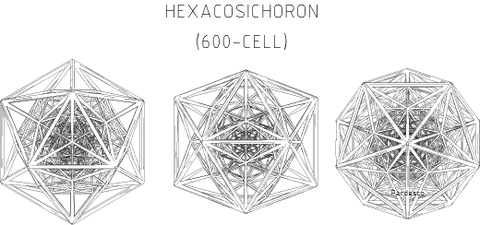The 600-cell consists of 600 tetrahedral cells. It is the four dimensional analogue of the icosahedron, since it has five tetrahedra meeting at every edge - just as the icosahedron has five triangles meeting at every vertex.

Below is a 600-cell performing a simple rotation in 3DDetails of the 600-cell (tetraplex)

• 600 cells
• 1200 faces
• 720 edges
• 120 vertices

600-cell Model (Interactive)

### 120-cell (dodecaplex)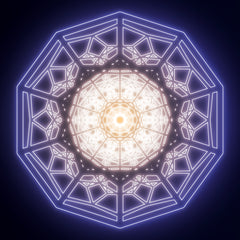120-cell, Pardesco, 2023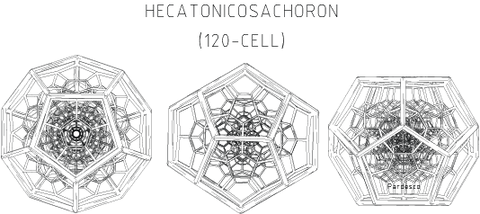The 120-cell also known as the dodecaplex or hyper-dodecahedron is composed of 120 dodecahedral cells. It is the four dimensional analogue of the dodecahedron. It is the sixth and largest of the regular 4-polytopes, having its predecessors inscribed within its geometries. It also has 120 instances of the 5-cell which is not found in any of the other regular polytopes.

Below is a 120-cell performing simple rotation in 3DDetails of the 120-cell (dodecaplex)

• 120 cells
• 720 faces
• 1200 edges
• 600 vertices

120-cell 3D Model (Interactive)

## Uniform Polychora and Other 4D Shapes"The fourth dimension is an extension of the other three into a higher realm of possibilities." - Max Tegmark

How are four dimensional shapes categorized?

Four-dimensional shapes can be categorized in a number of different ways, depending on the specific properties and characteristics of the shapes being considered. Some possible categories for four-dimensional shapes include:

Manifolds: A manifold is a topological space that locally resembles Euclidean space. Four-dimensional manifolds are higher-dimensional spaces that have properties that are similar to those of four-dimensional Euclidean space, but may have more complicated global structures.

Complexes: A complex is a higher-dimensional shape that is composed of a collection of lower-dimensional shapes, such as points, lines, triangles, and so on. Four-dimensional complexes can be very complex and can have many different types of symmetries and other interesting properties.

### Polytopes:

Scaliform polytope: A scaliform polytope is an isogonal polytope that can be represented with only one edge length, without the consideration that the elements themselves need to be uniform.

Uniform polytope: A uniform polytope of dimension three or higher is a vertex-transitive polytope bounded by uniform facets. The uniform polytopes in two dimensions are the regular polygons. (Uniform polytopes include the regular polytopes.) A strict definition requires uniform polytopes to be finite... a more expansive definition allows uniform honeycombs (2-dimensional tilings and higher dimensional honeycombs) of Euclidean and hyperbolic space to be considered polytopes as well.

Uniform honeycombs: a convex uniform honeycomb is a uniform tessellation which fills three-dimensional Euclidean space with non-overlapping convex uniform polyhedral cells.

### Hyperbolic Space:

Hyperbolic Space: In mathematics, hyperbolic space of dimension n is the unique simply connected, n-dimensional Riemannian manifold of constant sectional curvature equal to -1. It is homogeneous, and satisfies the stronger property of being a symmetric space

Uniform Honeycombs in hyperbolic space: a uniform honeycomb in hyperbolic space is a uniform tessellation of uniform polyhedral cells.

Below is a perspective projection of a dodecahedral tessellation in a hyperbolic 3-manifold. Four dodecahedra meet at each edge and eight at each vertex (like  in a cubic tessellation)### 4D Geometry Artwork by Pardesco

If you are interested in exploring the world of 4D geometry and supporting the work of artist Pardesco, consider purchasing one of their stunning 4D geometry artworks. These pieces are not only visually striking, but also offer a unique opportunity to experience the beauty and complexity of 4D space.

By purchasing a piece of 4D geometry artwork by Pardesco, you can not only bring a unique and thought-provoking piece into your home or office, but also support the work of a talented and dedicated artist. So don't miss out on this opportunity to own a truly one-of-a-kind piece of art. Visit Pardesco's website today to view their 4D geometry artworks and add one to your collection.

120-cell Technical Drawing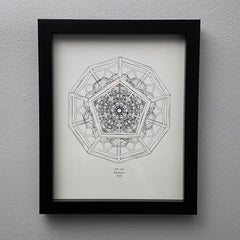600-cell Technical Drawing
120-cell Sculpture
600-cell Sculpture